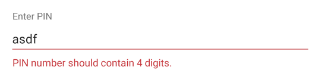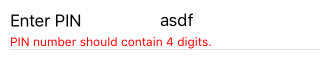#### NativeScript Core

If you followed the getting started section, you now know how to edit an object's properties with `RadDataForm` for NativeScript. From the validation overview you should have become acquainted with the validation feature in `RadDataForm`. This article will present you with the validators that you can use to validate your fields.

All validators have an `errorMessage` property which allows you to change the message that is displayed below the field when it is not valid.

## NonEmptyValidator

The `NonEmptyValidator` can be used with fields that are required so that empty input is not accepted as a valid value.

#### Figure 1: The `NonEmptyValidator` on Android (left) and iOS (right)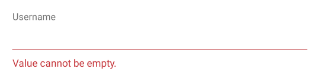## RangeValidator

The `RangeValidator` can be used with numeric values to determine if they fall within a specified range. The range is defined with the `minimum` and `maximum` properties.

#### Figure 2: The `RangeValidator` on Android (left) and iOS (right)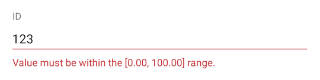## MinimumLengthValidator

The `MinimumLengthValidator` can be used with text values to determine whether the length of the current input has at least the length specified by the `length` property.

#### Figure 3: The `MinimumLengthValidator` on Android (left) and iOS (right)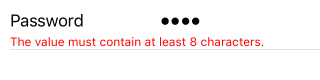## MaximumLengthValidator

The `MaximumLengthValidator` can be used with text values to determine whether the length of the current input is shorter or equal to the value specified with the `length` property.

#### Figure 4: The `MaximumLengthValidator` on Android (left) and iOS (right)## EmailValidator

The `EmailValidator` can be used with text values to determine whether the current input looks like an email. The email is defined with the following regular expression: `[a-zA-Z0-9\\+\\.\\_\\%\\-\\+]{1,256}\\@[a-zA-Z0-9][a-zA-Z0-9\\-]{0,64}(\\.[a-zA-Z0-9][a-zA-Z0-9\\-]{0,25})+`. If you want to use different criteria for matching an email, you can use the `RegExValidator` and specify your preferred regular expression.

#### Figure 5: The `EmailValidator` on Android (left) and iOS (right)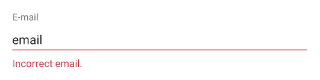## PhoneValidator

The `PhoneValidator` can be used with text values to determine whether the current input looks like a phone number. The phone number is defined with the following regular expression: `(\\+[0-9]+[\\- \\.]*)?(\\([0-9]+\\)[\\- \\.]*)?([0-9][0-9\\- \\.]+[0-9])`. If you want to use different criteria for matching a phone number, you can use the `RegExValidator` and specify your preferred regular expression.

#### Figure 6: The `PhoneValidator` on Android (left) and iOS (right)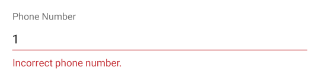## IsTrueValidator

The `IsTrueValidator` can be used with boolean values to determine whether the current input is positive. For example, this can be used to verify that the user has confirmed agreeing with some list with terms and conditions.

#### Figure 7: The `IsTrueValidator` on Android (left) and iOS (right)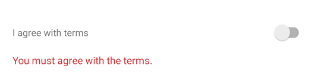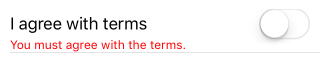## RegExValidator

The `RegExValidator` can be used with text values to determine whether the current input matches the regular expression given as value of the `regEx` property of the validator. Here's an example that validates that the input contains exactly 4 digits:

#### Example 1: Use the RegExValidator to validate if input contains 4 digits

``````<df:EntityProperty name="pin" displayName="Enter PIN" index="5">
<df:EntityProperty.validators>
<df:RegExValidator regEx="\d{4}" errorMessage="PIN number should contain 4 digits."/>
</df:EntityProperty.validators>
</df:EntityProperty>
``````

#### Figure 8: The `RegExValidator` on Android (left) and iOS (right)